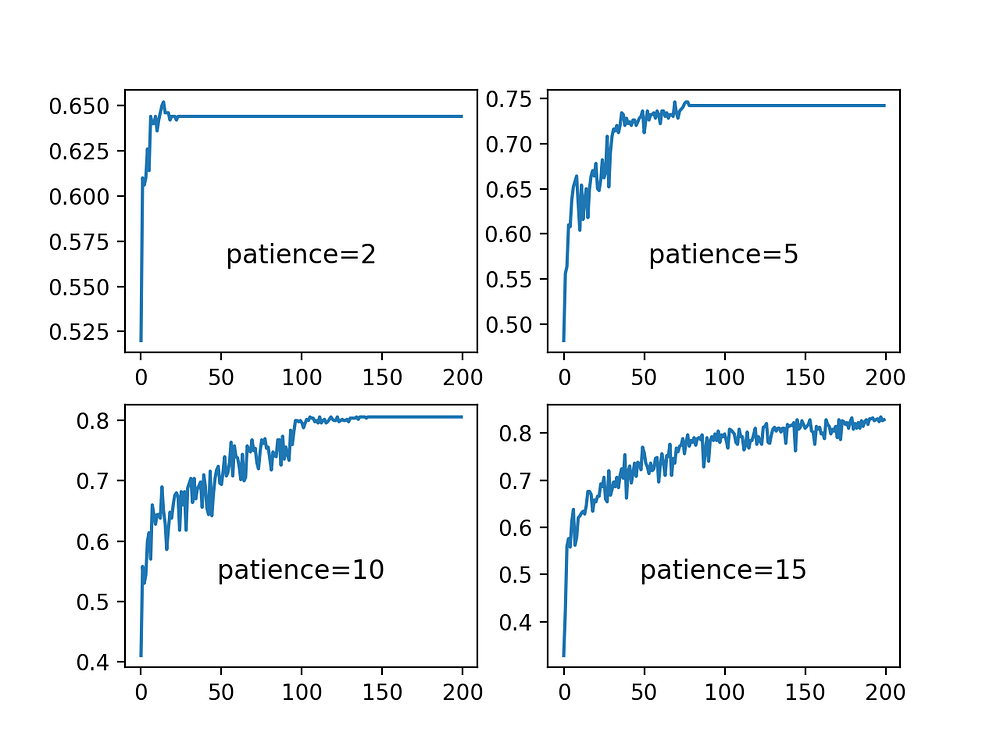# Is your epochs not performing? Try callbacks!

Source: Deep Learning on Medium# Is your epochs not performing? Try callbacks!

When we train a Neural Network, how much deep or shallow it is, we provide a parameter which tells how many times the data given should be seen again and again so that the loss function can be minimised perfectly. This parameter is called as ‘epochs’. After every epoch the Loss Function is minimized using Gradient Descent approaches. But, it is not necessary that more number of epochs will definitely mean that the loss function is going to be minimized. If that is the scenario, and we have given suppose 1000 epochs and the convergence of Loss Function stops at 50th epoch, your model will be running for 950 times unnecessarily. This will consume a lot of time, without helping us in accuracy.

Therefore, there are some approaches that can be used to tackle these situations. Keras provides one such functionality with the name of Callbacks. In this section we will be seeing two callbacks, out of a lot many: EarlyStopping and ReduceLRonPlateau.

Using EarlyStopping we can stop further epochs from running if we have seen that for some time the Loss is not reducing. But, we can also use ReduceLRonPlateau which before applying the EarlyStopping callback, will be used to decrease the learning rate by some factor, if the loss is not decreasing for some time. This may start the loss to decrease at a lesser Learning rate. There may be a possibility that still the loss may not decrease. This may lead to again executing the learning rate reduction, or may eventually lead to Early Stopping.

Let us look at the application of this on classifying Bank Credit data.

`import pandas as pddata1 = pd.read_csv(“Data/Dataset/BankCreditCard.csv”)`

Let us transform the data and create training and test sets

`from sklearn.model_selection import train_test_splitfrom sklearn.preprocessing import RobustScalerdata1.drop([‘Customer ID’], axis=1, inplace=True)X = data1.drop([‘Default_Payment’], axis=1)Y = data1[[‘Default_Payment’]]ss = RobustScaler()X = ss.fit_transform(X)X_train, X_test, Y_train, Y_test = train_test_split(X,Y, test_size=0.3, random_state=5)`

Let us define the callbacks now.

`from keras.callbacks import Callback, ReduceLROnPlateau, EarlyStoppingreduce_lr = ReduceLROnPlateau(monitor=’val_loss’, factor=0.1, patience=5, min_lr=1e-6, verbose=1)early_stop = EarlyStopping(monitor=’val_loss’, min_delta=0, patience=10, mode=’auto’)`

Let us create the Neural Network:

`model=Sequential()model.add(Dense(1024, input_dim=X_train.shape, activation=’tanh’))model.add(Dropout(0.5))model.add(BatchNormalization())model.add(Dense(512, activation=’tanh’))model.add(Dropout(0.4))model.add(BatchNormalization())model.add(Dense(256, activation=’tanh’))model.add(Dropout(0.3))model.add(BatchNormalization())model.add(Dense(128, activation=’tanh’))model.add(BatchNormalization())model.add(Dropout(0.2))model.add(Dense(32, activation=’tanh’))model.add(Dense(1, activation=’sigmoid’))adam = keras.optimizers.Adam(lr=5e-4,clipnorm=1.4)model.compile(loss=”binary_crossentropy”, optimizer=adam, metrics=[‘accuracy’, ‘mse’])model.fit(X_train, Y_train, validation_split=0.3, epochs=100, batch_size=64, callbacks=[reduce_lr, early_stop])`

The training will start now. A glimpse of which is given below: